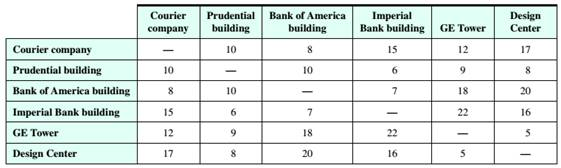# Route Planning A bike messenger needs to deliver packages to five different buildings and return to the courier company. The estimated biking times (in minutes) between the buildings are given in the following table. Use the greedy algorithm and the edge-picking algorithm to find two possible routes for the messenger to follow that Will help minimize the total travel time.### Mathematical Excursions (MindTap C...

4th Edition
Richard N. Aufmann + 3 others
Publisher: Cengage Learning
ISBN: 9781305965584

#### Solutions

Chapter
Section### Mathematical Excursions (MindTap C...

4th Edition
Richard N. Aufmann + 3 others
Publisher: Cengage Learning
ISBN: 9781305965584
Chapter 5.2, Problem 28ES
Textbook Problem
153 views

## Route Planning A bike messenger needs to deliver packages to five different buildings and return to the courier company. The estimated biking times (in minutes) between the buildings are given in the following table. Use the greedy algorithm and the edge-picking algorithm to find two possible routes for the messenger to follow that Will help minimize the total travel time.To determine

To find two possible routes, starting and ending at courier company, for the messenger to follow that will minimize his total travel time, using the greedy algorithm and the edge-picking algorithm.

### Explanation of Solution

Given information:

Given, a bike messenger needs to deliver packages to five different buildings and return to the courier company.

The estimated biking times (in minutes) between the buildings are given in the table below:

Calculation:

Given, there are five locations to deliver and the messenger starts and ends at Courier Company.

Hence six vertices corresponding to the six locations are marked with respective names.

And then making the complete graph by marking every possible edge with every edge marked with the weight represented in the table between the locations.

The biking time between Courier Company and Prudential building is 10. Hence the edge drawn between them has a weight of 10.

Similarly marking all the edges with respective weights, the graph is given as:

Greedy algorithm:

To use the greedy algorithm, the graph must be Hamiltonian.

A path in a Hamiltonian graph is said to be a Hamiltonian Circuit if it begins and ends at the same vertex and passes through each vertex of a graph exactly once.

The greedy algorithm states to travel along a connected edge that has the smallest weight that is not yet visited. This process continues till all the vertices are visited and returned to the starting vertex.

Here, the vertex representing Courier Company is selected.

Vertex representing Courier Company is of degree 5 and the weights of the connected edges are 10, 8, 15, 12, 17

Hence, as per the Greedy Algorithm the edge with weight 8 is selected i.e. path reaches vertex representing Bank of America Building.

Vertex representing Bank of America Building is of degree 5 and the weights of the connected edges are 8, 10, 7, 18, 20

Hence, as per the Greedy Algorithm the edge with weight 7 is selected i.e. path reaches vertex representing Imperial Bank Building.

Vertex representing Imperial Bank Building is of degree 5 and the weights of the connected edges are 15, 6, 7, 22, 16

Hence, as per the Greedy Algorithm the edge with weight 6 is selected i.e. path reaches vertex representing Prudential Building.

Vertex representing Prudential Building is of degree 5 and the weights of the connected edges are 10, 10, 6, 9, 8

The least of the weights is 6 but it leads to vertex representing Imperial Bank Building which is already visited.

Hence, as per the Greedy Algorithm the edge with weight 8 is selected i.e. path reaches vertex representing Design Center.

Vertex representing Design Center is of degree 5 and the weights of the connected edges are 17, 8, 20, 16, 5

Hence, as per the Greedy Algorithm the edge with weight 5 is selected i.e. path reaches vertex representing GE Tower.

Vertex representing GE Tower is of degree 5 and the weights of the connected edges are 12, 9, 18, 22, 5

The least of the weights is 5 but it leads to vertex representing GE Tower which is already visited. The next least of the weights is 9 but it leads to vertex representing Prudential Building which is already visited.

Hence, as per the Greedy Algorithm the edge with weight 12 is selected i...

### Still sussing out bartleby?

Check out a sample textbook solution.

See a sample solution

#### The Solution to Your Study Problems

Bartleby provides explanations to thousands of textbook problems written by our experts, many with advanced degrees!

Get Started

Find more solutions based on key concepts
For Problems 11-18, evaluate each expression. 44211102

Mathematical Applications for the Management, Life, and Social Sciences

Find an equation of the line that passes through the point (3, 2) and is parallel to the line passing through t...

Applied Calculus for the Managerial, Life, and Social Sciences: A Brief Approach

Find f. f(x) = ex 2 sin x, f(0) = 3, f(/2) = 0

Single Variable Calculus: Early Transcendentals, Volume I

Describe the set of points (x,y) such that x2+y2=0.

Finite Mathematics and Applied Calculus (MindTap Course List)

True or False: In the definition of , the expression is interpreted as “the distance between f(x) and L is les...

Study Guide for Stewart's Single Variable Calculus: Early Transcendentals, 8th

What Goes Up (a) It is well known that the model in which air resistance is ignored, part (a) of Problem 36, p...

A First Course in Differential Equations with Modeling Applications (MindTap Course List)

Used Car Prices. According to the National Automobile Dealers Association, the mean price for used cars is \$10,...

Modern Business Statistics with Microsoft Office Excel (with XLSTAT Education Edition Printed Access Card) (MindTap Course List)

TEST YOUR UNDERSTANDING In the situation of the example, if one explosion releases triple the energy of another...

Functions and Change: A Modeling Approach to College Algebra (MindTap Course List)